Effective at SESYNC's closure in December 2022, this page is no longer maintained. The information may be out of date or inaccurate.

# Introduction to Geospatial Data

Handouts for this lesson need to be saved on your computer. Download and unzip this material into the directory (a.k.a. folder) where you plan to work.

## Lesson Objectives

• Meet the key R packages for geospatial analysis
• Learn basic wrangling of vector and raster data

## Specific Achievements

• Create, load, and plot vector data types, or “features”
• Filter features based on associated data or on spatial location
• Perform geometric operations on polygons
• Load, manipulate, and extract raster data

Top of Section

## R Packages

The key R packages for this lesson are

### OSGeo Dependencies

Most R packages depend on other R packages. The sf and stars packages also depend on system libraries.

• GDAL for read/write in geospatial data formats
• GEOS for geometry operations
• PROJ for cartographic projections

System libraries cannot be installed by R’s install.packages(), but can be bundled with these packages and for private use by them. Either way, the necessary libraries are maintained by the good people at the Open Source Geospatial Foundation for free and easy distribution. When you load sf, it will return an error if any dependencies are not found.

### Vector Data

The US Census website distributes county polygons (and much more) that are provided with the handouts. The sf package reads shapefiles (“.shp”) and most other vector data:

library(sf)

shp <- 'data/cb_2016_us_county_5m'


The object shp is a character string with the location of a folder that contains the collection of individual files that make up a shapefile.

The counties object is a data.frame that includes a sfc, which stands for “simple feature column”. This special column is usually called “geometry.”

> head(counties)

Simple feature collection with 6 features and 9 fields
Geometry type: MULTIPOLYGON
Dimension:     XY
Bounding box:  xmin: -114.7556 ymin: 29.26116 xmax: -81.10192 ymax: 38.77443
STATEFP COUNTYFP COUNTYNS       AFFGEOID GEOID      NAME LSAD       ALAND
1      04      015 00025445 0500000US04015 04015    Mohave   06 34475567011
2      12      035 00308547 0500000US12035 12035   Flagler   06  1257365642
3      20      129 00485135 0500000US20129 20129    Morton   06  1889993251
4      28      093 00695770 0500000US28093 28093  Marshall   06  1828989833
5      29      510 00767557 0500000US29510 29510 St. Louis   25   160458044
6      35      031 00929107 0500000US35031 35031  McKinley   06 14116799068
AWATER                       geometry
1 387344307 MULTIPOLYGON (((-114.7556 3...
2 221047161 MULTIPOLYGON (((-81.52366 2...
3    507796 MULTIPOLYGON (((-102.042 37...
4   9195190 MULTIPOLYGON (((-89.72432 3...
5  10670040 MULTIPOLYGON (((-90.31821 3...
6  14078537 MULTIPOLYGON (((-109.0465 3...


In this case, we have a MULTIPOLYGON object which means that each row has a column with information about the boundaries of a county polygon.

### Geometry Types

Like any data.frame column, the geometry column is comprised of a single data type. The “MULTIPOLYGON” is just one of several standard geometric data types.

Common Types Description
POINT zero-dimensional geometry containing a single point
LINESTRING sequence of points connected by straight, non-self intersecting line pieces; one-dimensional geometry
POLYGON sequence of points in closed, non-intersecting rings; the first denotes the exterior ring, any subsequent rings denote holes
MULTI* set of * (POINT, LINESTRING, or POLYGON)

The spatial data types are built upon each other in a logical way: lines are built from points, polygons are built from lines, and so on.

We can create any of these spatial objects from coordinates. Here’s an sfc object with a single “POINT”, corresponding to SESYNC’s position in degrees latitude and degrees longitude, with the same coordinate reference system as the counties object.

sesync <- st_sfc(st_point(
c(-76.503394, 38.976546)),
crs = st_crs(counties))


### Coordinate Reference Systems

A key feature of a geospatial data type is its associated CRS, stored in Well Known Text (WKT) format. We can print the CRS of a spatial object with st_crs().

> st_crs(counties)

Coordinate Reference System:
wkt:
DATUM["North American Datum 1983",
ELLIPSOID["GRS 1980",6378137,298.257222101,
LENGTHUNIT["metre",1]]],
PRIMEM["Greenwich",0,
ANGLEUNIT["degree",0.0174532925199433]],
CS[ellipsoidal,2],
AXIS["latitude",north,
ORDER,
ANGLEUNIT["degree",0.0174532925199433]],
AXIS["longitude",east,
ORDER,
ANGLEUNIT["degree",0.0174532925199433]],
ID["EPSG",4269]]


The WKT contains parameters of the projection. The last line includes the EPSG ID, a numerical code indicating the coordinate reference system.

In order for two spatial objects to be comparable, they must be represented in the same coordinate reference system. This can be a geographic coordinate system or a projected coordinate system.

Geographic coordinate systems, like the one we saw when we called st_crs(counties), represent locations as degrees of latitude and longitude on the surface of the Earth, modeled as an ellipsoid (a slightly flattened sphere). The datum of a geographic coordinate system is the set of parameters representing the exact shape of the ellipsoid. The counties object uses the 1983 North American Datum, or NAD83 for short. Most world maps use the WGS84 datum.

A projected coordinate system converts geographical coordinates into Cartesian or rectangular (X, Y) coordinates for making two-dimensional maps. Since it is impossible to provide an exact two-dimensional representation of a three-dimensional object like Earth without losing some information, specialized projections were developed for different regions of the world and different analytical applications. For example, the Mercator projection (left) preserves shapes and angles, which is useful for navigation, but it greatly inflates areas at the poles. The Lambert equal-area projection (right) preserves areas at the cost of shape distortion away from its center.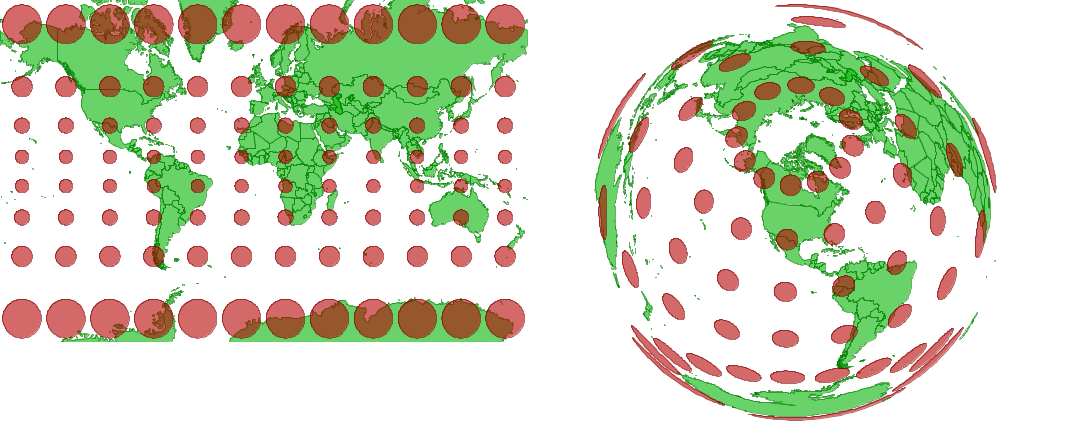Projections can preserve shapes or areas, but not both.

Source

### Bounding Box

A bounding box for all features in an sf data frame is generated by st_bbox().

> st_bbox(counties)

      xmin       ymin       xmax       ymax
-179.14734  -14.55255  179.77847   71.35256


The bounding box is not a static attribute—it is determined on-the-fly for the entire table or any subset of features. In this example, we subset the United States counties by state ID 24 (Maryland).

Because the counties object is a kind of data.frame, we can use dplyr verbs such as filter on it, just as we would with a non-spatial data frame. After filtering, you can see that the bounding box is now much smaller.

library(dplyr)
counties_md <- filter(
counties,
STATEFP == '24')

> st_bbox(counties_md)

     xmin      ymin      xmax      ymax
-79.48765  37.91172 -75.04894  39.72312


### Grid

A bounding box summarizes the limits, but is not itself a geometry (not a POINT or POLYGON), even though it has a CRS attribute.

> st_crs(st_bbox(counties_md))

Coordinate Reference System:
wkt:
DATUM["North American Datum 1983",
ELLIPSOID["GRS 1980",6378137,298.257222101,
LENGTHUNIT["metre",1]]],
PRIMEM["Greenwich",0,
ANGLEUNIT["degree",0.0174532925199433]],
CS[ellipsoidal,2],
AXIS["latitude",north,
ORDER,
ANGLEUNIT["degree",0.0174532925199433]],
AXIS["longitude",east,
ORDER,
ANGLEUNIT["degree",0.0174532925199433]],
ID["EPSG",4269]]


We can use st_make_grid() to make a rectangular grid over a sf object. The grid is a geometry—by default, a POLYGON.

grid_md <- st_make_grid(counties_md,
n = 4)


The st_make_grid() function draws a rectangular grid on the surface of a sphere. At the relatively small spatial scale of the state of Maryland, the difference between a flat surface and the surface of a sphere is minimal.

> grid_md

Geometry set for 16 features
Geometry type: POLYGON
Dimension:     XY
Bounding box:  xmin: -79.48765 ymin: 37.91172 xmax: -75.04894 ymax: 39.72312
First 5 geometries:

POLYGON ((-79.48765 37.91172, -78.37797 37.9117...

POLYGON ((-78.37797 37.91172, -77.26829 37.9117...

POLYGON ((-77.26829 37.91172, -76.15862 37.9117...

POLYGON ((-76.15862 37.91172, -75.04894 37.9117...

POLYGON ((-79.48765 38.36457, -78.37797 38.3645...


### Plot Layers

Spatial objects defined by sf are compatible with the plot function. Setting the plot parameter add = TRUE allows an existing plot to serve as a layer underneath the new one. The two layers should have the same coordinate reference system.

The following code plots the grid first, then the ALAND (land area) column of counties_md. This plots the county boundaries with the fill color of the polygons corresponding to the area of the polygon. The default color scheme means that larger counties appear yellow. Finally, we overlay the point location of SESYNC.

plot(grid_md)
plot(counties_md['ALAND'],
plot(sesync, col = "green",
pch = 20, add = TRUE)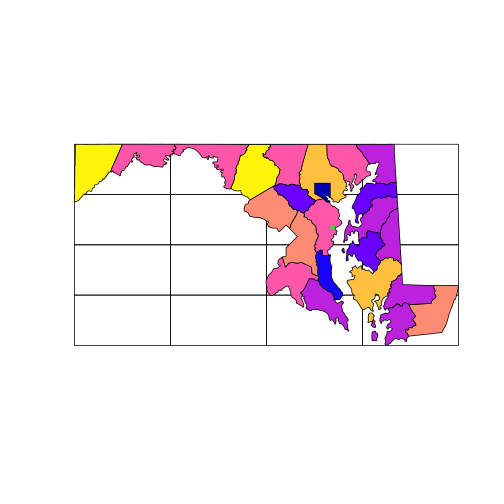But note that the plot function won’t prevent you from layering up geometries with different coordinate systems: you must safeguard your own plots from this mistake. The arguments col and pch, by the way, are graphical parameters used in base R, see ?par.

### Customizing maps with ggplot2

The plot function is great for quick visualizations but the ggplot2 package gives you much more flexibility to customize your plots.

For those not familiar with ggplot2, a comprehensive introduction can be found in the Data Visualisation chapter of R for Data Science by Wickham and Grolemund, or check out SESYNC’s introductory ggplot2 lesson.

Producing any type of graph with ggplot2 requires a similar sequence of steps:

• Begin by calling ggplot() and add any further plot elements by “adding” them with +;
• Specify the dataset as well as aesthetic mappings (aes), which associate variables in the data to graphical elements (x and y axes, color, size and shape of points, etc.);
• Add geom_ layers, which specify the type of graph;
• Optionally, specify additional customization options such as axis names and limits, color themes, and more.

Let’s plot the Maryland county map with ggplot().

All sf objects can be plotted by adding geom_sf() layers to the plot, which automatically associates the geometry column with the x and y coordinates of the features in the correct projection. If there are multiple geom_sf() layers, ggplot() will transform all layers to the projection system of the first layer added to the plot.

library(ggplot2)

ggplot() +
geom_sf(data = counties_md,
aes(fill = ALAND)) +
geom_sf(data = sesync,
size = 3, color = 'red')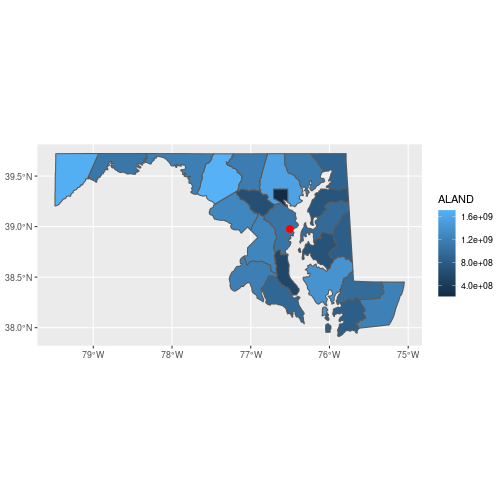You can customize the plot theme and color scales, among other things.

theme_set(theme_bw())

ggplot() +
geom_sf(data = counties_md,
aes(fill = ALAND/1e6), color = NA) +
geom_sf(data = sesync,
size = 3, color = 'red') +
scale_fill_viridis_c(name = 'Land area (sq. km)') +
theme(legend.position = c(0.3, 0.3))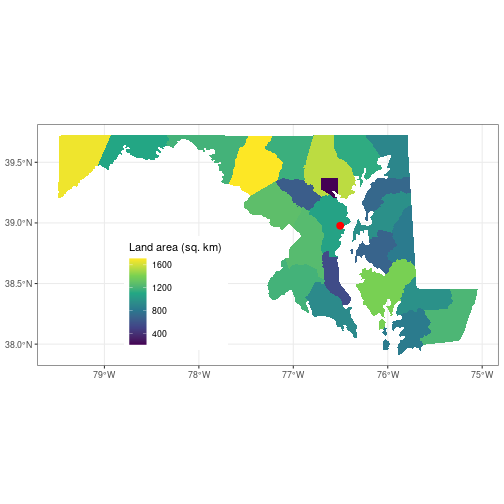Here, we first set the plot theme for all other ggplot() plots we create in this session to a black-and-white theme to get rid of the gray background. Next, we divide the land area value by 1e6 to convert it to square km, then specify color = NA for the county polygons so there is no border drawn around them. We add a colorblind-friendly viridis fill scale for the polygons and add an understandable legend title with the name argument to the fill scale. Finally we move the legend to coordinates c(0.3, 0.3) in the panel (the legend position can range from 0 to 1 on both axes). In all cases we add additional customization elements to the base plot using +.

### Spatial Subsetting

An object created with st_read() is a data.frame, which is why the dplyr function filter used above on the non-geospatial column named STATEFP worked normally. The function st_filter() does the equivalent of a filtering operation on the geometry column, known as a spatial “overlay.”

> st_filter(counties_md, sesync)

Simple feature collection with 1 feature and 9 fields
Geometry type: MULTIPOLYGON
Dimension:     XY
Bounding box:  xmin: -76.84036 ymin: 38.71356 xmax: -76.39408 ymax: 39.2374
STATEFP COUNTYFP COUNTYNS       AFFGEOID GEOID         NAME LSAD      ALAND
1      24      003 01710958 0500000US24003 24003 Anne Arundel   06 1074553083
AWATER                       geometry
1 447833714 MULTIPOLYGON (((-76.84036 3...


st_filter subsets the features of a sf object based on spatial (rather than numeric or string) matching. Matching is implemented with functions like st_within(x, y). Here we filtered the counties_md object to retain only the features that intersect with the single point contained in the sesync object. Because sesync is a single point, this results in a sfc with a single row.

The overlay functions in the sf package follow the pattern st_predicate(x, y) and perform the test “x [is] predicate y”. Some key examples are:

 st_intersects boundary or interior of x intersects boundary or interior of y st_within interior and boundary of x do not intersect exterior of y st_contains y is within x st_overlaps interior of x intersects interior of y st_equals x has the same interior and boundary as y

### Coordinate Transforms

For the next part of this lesson, we import a new polygon layer corresponding to the 1:250k map of US hydrological units (HUC) downloaded from the United States Geological Survey.

shp <- 'data/huc250k'


Compare the coordinate reference systems of counties and huc by looking at the WKT of both objects.

> st_crs(counties_md)

Coordinate Reference System:
wkt:
DATUM["North American Datum 1983",
ELLIPSOID["GRS 1980",6378137,298.257222101,
LENGTHUNIT["metre",1]]],
PRIMEM["Greenwich",0,
ANGLEUNIT["degree",0.0174532925199433]],
CS[ellipsoidal,2],
AXIS["latitude",north,
ORDER,
ANGLEUNIT["degree",0.0174532925199433]],
AXIS["longitude",east,
ORDER,
ANGLEUNIT["degree",0.0174532925199433]],
ID["EPSG",4269]]

> st_crs(huc)

Coordinate Reference System:
wkt:
DATUM["North American Datum 1927",
ELLIPSOID["Clarke 1866",6378206.4,294.9786982,
LENGTHUNIT["metre",1]],
ID["EPSG",6267]],
PRIMEM["Greenwich",0,
ANGLEUNIT["Degree",0.0174532925199433]]],
CONVERSION["unnamed",
METHOD["Albers Equal Area",
ID["EPSG",9822]],
PARAMETER["Latitude of false origin",23,
ANGLEUNIT["Degree",0.0174532925199433],
ID["EPSG",8821]],
PARAMETER["Longitude of false origin",-96,
ANGLEUNIT["Degree",0.0174532925199433],
ID["EPSG",8822]],
PARAMETER["Latitude of 1st standard parallel",29.5,
ANGLEUNIT["Degree",0.0174532925199433],
ID["EPSG",8823]],
PARAMETER["Latitude of 2nd standard parallel",45.5,
ANGLEUNIT["Degree",0.0174532925199433],
ID["EPSG",8824]],
PARAMETER["Easting at false origin",0,
LENGTHUNIT["metre",1],
ID["EPSG",8826]],
PARAMETER["Northing at false origin",0,
LENGTHUNIT["metre",1],
ID["EPSG",8827]]],
CS[Cartesian,2],
AXIS["(E)",east,
ORDER,
LENGTHUNIT["metre",1,
ID["EPSG",9001]]],
AXIS["(N)",north,
ORDER,
LENGTHUNIT["metre",1,
ID["EPSG",9001]]]]


The Census data uses unprojected (longitude, latitude) coordinates, but huc is in an Albers equal-area projection (indicated as PROJECTION["Albers_Conic_Equal_Area"]). Maps of the continental United States often use the Albers projection.

Important Note: Until recently, coordinate systems were represented with PROJ.4 strings, but in 2020 the developers of sf replaced them with the more precise Well Known Text format. We will still use PROJ.4 strings in this lesson to transform coordinate systems. Keep in mind, though, that development of geospatial R packages is always ongoing, and this lesson may be updated in the future to reflect that development.

The function st_transform() converts an sfc between coordinate reference systems, specified with the parameter crs = x. A numeric x must be a valid EPSG code; a character x is interpreted as a PROJ.4 string.

For example, the unprojected CRS of counties can be represented as the PROJ.4 string "+proj=longlat +datum=NAD83 +no_defs". This character string is a list of parameters that correspond to the EPSG code 4269. You can transform objects to that projection using the PROJ.4 string as the argument to crs, or by typing crs = 4269. The EPSG code or PROJ.4 string is translated to the WKT format.

Not all projections have an EPSG code. The projection in the PROJ.4 string below does not have an EPSG code, for example. We’ll transform all the sfc objects to this projection because it is the one used by the NLCD raster layer we’ll introduce later in this lesson.

prj <- '+proj=aea +lat_1=29.5 +lat_2=45.5 \
+lat_0=23 +lon_0=-96 +x_0=0 +y_0=0 \
+datum=WGS84 +units=m +no_defs'


PROJ.4 strings contain a reference to the type of projection—this one is another Albers equal-area, as indicated by +proj=aea—along with numeric parameters associated with that projection. An additional important parameter that may differ between two coordinate systems is the “datum”, which indicates the standard by which the irregular surface of the Earth is approximated by an ellipsoid in the coordinates themselves.

Use st_transform() to assign the two layers and SESYNC’s location to a common projection string (prj). This takes a few moments, as it recalculates coordinates for every vertex in the sfc.

counties_md <- st_transform(
counties_md,
crs = prj)
huc <- st_transform(
huc,
crs = prj)
sesync <- st_transform(
sesync,
crs = prj)


Now that the three objects share a projection, we can plot the county boundaries, watershed boundaries, and SESYNC’s location on a single map.

plot(st_geometry(counties_md))
plot(st_geometry(huc),
border = 'blue', add = TRUE)
plot(sesync, col = 'green',
pch = 20, add = TRUE)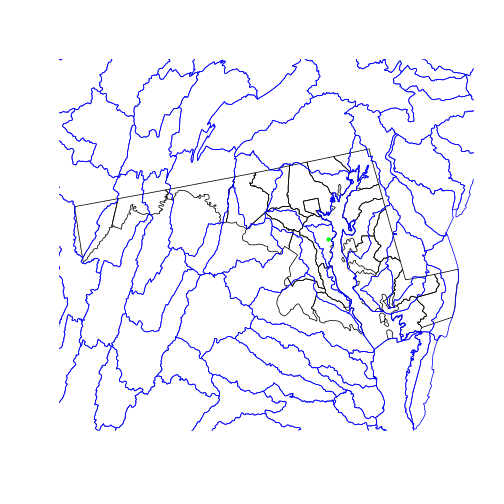We use the st_geometry() function on the two polygon layers we plot, which only plots the polygon boundaries (the geometry column) rather than filling the areas with colors. st_geometry(x) is equivalent to x\$geometry.

### Geometric Operations

The data for a map of watershed boundaries within the state of MD is all here: in the country-wide huc and in the state boundary “surrounding” all of counties_md. To get just the HUCs inside Maryland’s borders:

• remove the internal county boundaries within the state
• clip the hydrological areas to their intersection with the state

The first step is a spatial union operation: we want the resulting object to combine the area covered by all the multipolygons in counties_md.

state_md <- st_union(counties_md)
plot(state_md)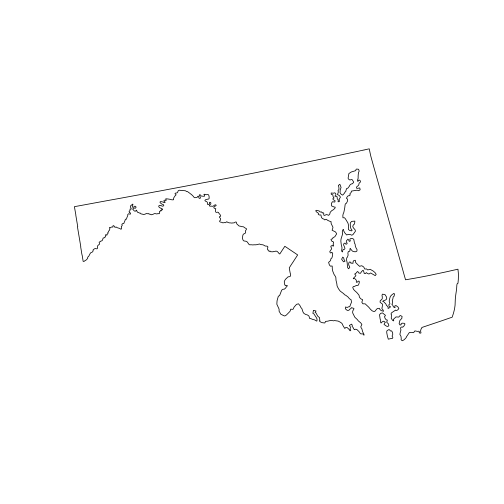To perform a union of all sub-geometries in a single sfc, we use the st_union() function with a single argument. The output, state_md, is a new sfc that is no longer a column of a data frame. Tabular data can’t safely survive a spatial union because the polygons corresponding to the attributes in the table have been combined into a single larger polygon. So the data frame columns are discarded.

The second step is a spatial intersection, since we want to limit the polygons to areas covered by both huc and state_md.

huc_md <- st_intersection(
huc,
state_md)

Warning: attribute variables are assumed to be spatially constant throughout all
geometries

plot(state_md)
plot(st_geometry(huc_md), border = 'blue',
col = NA, add = TRUE)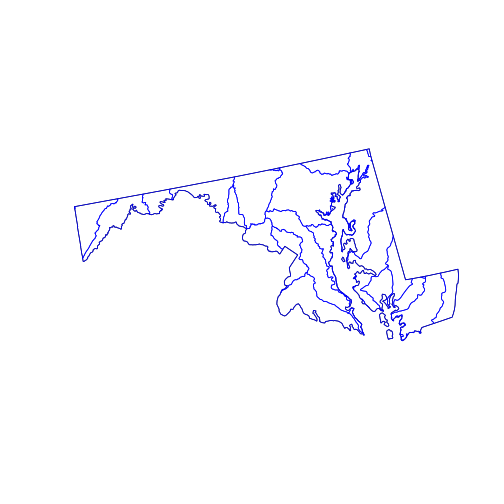The st_intersection() function intersects its first argument with the second. The individual hydrological units are preserved but any part of them (or any whole polygon) lying outside the state_md polygon is cut from the output. The attribute data remains in the corresponding records of the data.frame, but (as warned) has not been updated. For example, the “AREA” attribute of any clipped HUC does not reflect the area of the new, smaller polygon.

The sf package provides many functions dealing with distances and areas.

• st_buffer: to create a buffer of specific width around a geometry
• st_distance: to calculate the shortest distance between geometries
• st_area: to calculate the area of polygons

These functions were recently updated to use geodesic distances that account for Earth’s curvature, making them accurate even at large spatial scales. You might also want to check out the geosphere package.

Top of Section

## Raster Data

Raster data is a matrix or cube with additional spatial metadata (e.g. extent, resolution, and projection) that allows its values to be mapped onto geographical space. The stars package provides the read_stars() function for reading the many formats of such data.

In the past (from 2010 to 2020), the raster package was the primary R package for reading and working with raster data. Recently, the developers of sf released stars (Spatio-Temporal ARrayS). It is integrated more closely with sf and related packages, as well as with the “tidyverse” family of packages, and has significant performance advantages. Another new alternative is terra, created by the developer of raster. Older versions of this lesson use raster, and you may come across the raster package when searching for help on geospatial data analysis in R. Nevertheless we recommend terra or stars because of their simplicity and speed advantages, and also because they are more likely to be updated and maintained in the future.

The National Land Cover Database is a 30m resolution grid of cells classified as forest, crops, wetland, developed, etc., across the continental United States. The file provided in this lesson is cropped and reduced to a lower resolution in order to speed processing.

library(stars)
nlcd <- read_stars("data/nlcd_agg.tif", proxy = FALSE)
nlcd <- droplevels(nlcd)


Here we set proxy = FALSE because this is a small example raster that we can load into working memory. If proxy = TRUE, raster data is not loaded into working memory, as you can confirm by checking the R object size with object.size(nlcd). This means that unlike most analyses in R, you can actually process raster datasets larger than the RAM available on your computer; stars automatically loads pieces of the data and computes on each of them in sequence. By default, stars will load small rasters into working memory and large rasters as proxies unless you specify otherwise.

Also note that the nlcd_agg.tif file is accompanied by a metadata file called nlcd_agg.tif.aux.xml that stores information including descriptive names for the different land cover classes. We need to call droplevels(nlcd) to remove empty factor levels. The NLCD 2016 legend classification codes are not sequential and begin with 11, but factor levels in R begin with 1. Using droplevels gets rid of the empty levels so that the legends on the plots we will make in a moment do not include blank levels.

The default print method for a stars raster object is a summary of metadata contained in the raster file.

> nlcd

stars object with 2 dimensions and 1 attribute
attribute(s), summary of first 1e+05 cells:
nlcd_agg.tif
Deciduous Forest        :32576
Cultivated Crops        :13014
Developed, Open Space   :11063
Hay/Pasture             :10729
Mixed Forest            : 9260
Developed, Low Intensity: 8034
(Other)                 :15324
dimension(s):
from   to  offset delta                    refsys point values x/y
x    1 3004 1394535   150 Albers_Conical_Equal_Area FALSE   NULL [x]
y    1 2514 2101515  -150 Albers_Conical_Equal_Area FALSE   NULL [y]


The plot method interprets the pixel values of the raster matrix according to a pre-defined color scheme.

> plot(nlcd)

downsample set to c(5,5)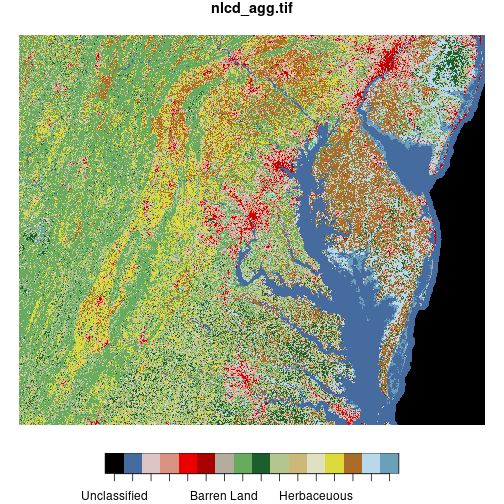The st_crop() function trims a stars raster object to the dimensions of a given spatial object or bounding box.

Here we crop the NLCD raster to the bounding box of the huc_md polygon, then display both layers on the same map. (We can plot polygon and raster layers together on the same map, just like we can plot multiple polygon layers—as long as they have the same coordinate reference system!) Because ggplot2 does not automagically recognize the predefined color scheme from the original image file, we have to extract the color scheme using attr(nlcd[], 'colors') and use it as an argument to scale_fill_manual().

md_bbox <- st_bbox(huc_md)
nlcd <- st_crop(nlcd, md_bbox)

ggplot() +
geom_stars(data = nlcd) +
geom_sf(data = huc_md, fill = NA) +
scale_fill_manual(values = attr(nlcd[], 'colors'))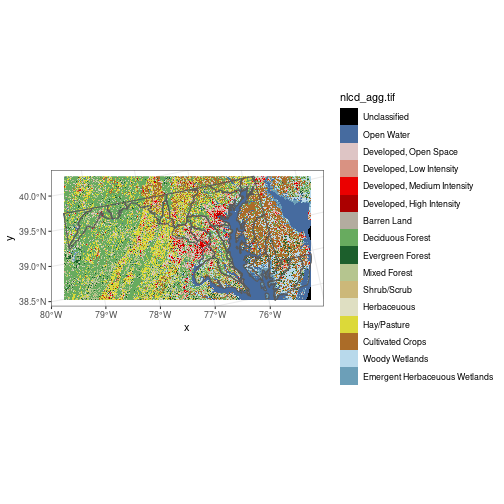A raster is fundamentally a data matrix, and individual pixel values can be extracted by regular matrix subscripting. The matrix of pixel values is found in list element [] of a stars object. For example, the value of the bottom-left corner pixel:

> nlcd[][1, 1]

 Herbaceuous
16 Levels: Unclassified Open Water ... Emergent Herbaceuous Wetlands


The index [1, 1] corresponds to the bottom left pixel because the raster represents a two-dimensional image with x and y coordinates. The origin of the axes is at the bottom left.

For this particular dataset, land cover class is represented as a factor variable. We can display the factor levels of nlcd[]:

> levels(nlcd[])

  "Unclassified"                  "Open Water"
 "Developed, Open Space"         "Developed, Low Intensity"
 "Developed, Medium Intensity"   "Developed, High Intensity"
 "Barren Land"                   "Deciduous Forest"
 "Evergreen Forest"              "Mixed Forest"
 "Shrub/Scrub"                   "Herbaceuous"
 "Hay/Pasture"                   "Cultivated Crops"
 "Woody Wetlands"                "Emergent Herbaceuous Wetlands"


Writing pull(nlcd) is equivalent to nlcd[]. This can be useful if you are extracting values from a raster as part of a “pipe.” For example, nlcd %>% pull %>% levels would give the same result as the code above.

### Raster Math

Mathematical functions called on a raster get applied to each pixel. For a single raster r, the function log(r) returns a new raster where each pixel’s value is the log of the corresponding pixel in r.

Likewise, addition with r1 + r2 creates a raster where each pixel is the sum of the values from r1 and r2, and so on. Naturally, spatial attributes of rasters (e.g. extent, resolution, and projection) must match for functions that operate pixel-wise on multiple rasters.

Logical operations work too: r1 > 5 returns a raster with pixel values TRUE or FALSE and is often used to “mask” a raster, or remove pixels that don’t meet a certain logical condition.

forest_types <- c('Evergreen Forest', 'Deciduous Forest', 'Mixed Forest')

downsample set to c(3,3)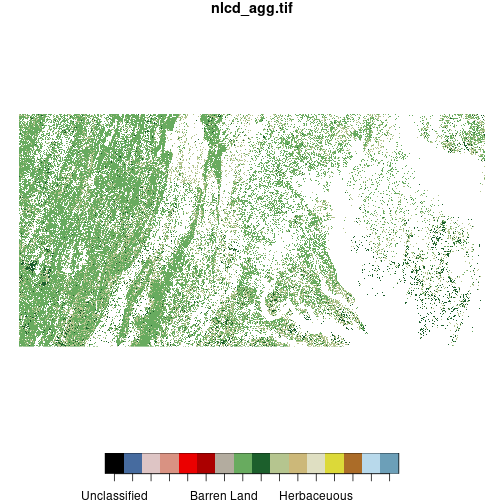We define a vector including the levels of nlcd representing three types of forest and create a copy of nlcd called forest_mask. We “mask out” all pixels in forest_mask that do not meet the logical condition forest %in% forest_types, setting them to NA. (The ! negates a logical condition.) This results in a raster where all pixels that are not classified as forest are removed.

To further reduce the resolution of the nlcd raster, the st_warp() function combines values in a block of a given size using a given method.

nlcd_agg <- st_warp(nlcd,
cellsize = 1500,
method = 'mode',
use_gdal = TRUE)

nlcd_agg <- droplevels(nlcd_agg)
levels(nlcd_agg[]) <- levels(nlcd[])

plot(nlcd_agg)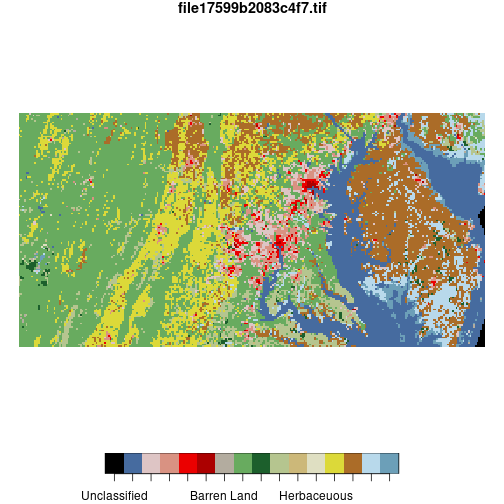Here, cellsize = 1500 specifies that the raster should be downsampled to 1500-meter pixel size. The input raster has 150-meter resolution, so this will convert 10-by-10 blocks of pixels to single pixels. The argument method = 'mode' indicates that the aggregated value is the mode of the input pixels making up each output pixel (averaging would not work since land cover is a categorical variable). use_gdal = TRUE specifies to use the GDAL system library “behind the scenes” to do the aggregation, which is needed for aggregation methods including mode. Unfortunately, the call to GDAL strips away the land cover class names so we replace them by dropping unused levels with droplevels() and then replacing the names with levels().

Top of Section

## Crossing Rasters with Vectors

Raster and vector geospatial data in R require different packages. The creation of geospatial tools in R has been a community effort, and not necessarily a well-organized one. Development is still ongoing on many packages dealing with raster and vector data. The stars package has not yet released a “version 1.0” (it’s at 0.5 as of this writing). Developers are working hard on improving the package but if you notice any issues or bugs, report them on their GitHub repo.

Although not needed in this lesson, you may notice when using some older geospatial packages that it is necessary to convert a vector object from sf (class beginning with sfc*) to sp (class beginning with Spatial*). sp is an older package that was replaced by sf, and many geospatial packages still work with sp objects. You can do this by calling sp_object <- as(sf_object, 'Spatial'). You may also need to convert a stars object to a Raster* class object, which you can do by calling stars_object <- as(sf_object, 'Raster').

The st_extract() function allows subsetting and aggregation of raster values based on a vector spatial object. For example we might want to extract the NLCD land cover class at SESYNC’s location.

plot(nlcd, reset = FALSE)

downsample set to c(3,3)

plot(sesync, col = 'green',
pch = 16, cex = 2, add = TRUE)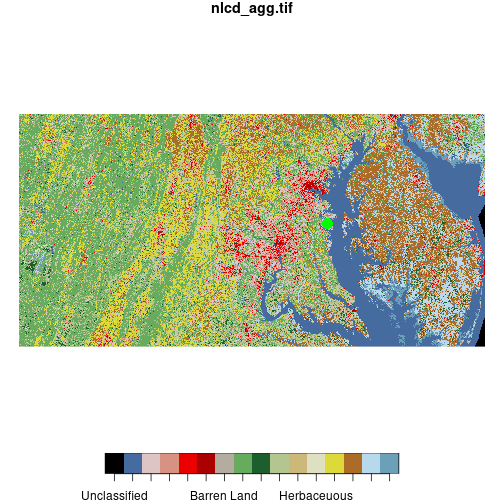When we use plot() to combine raster and vector layers we need to set reset = FALSE on the first layer we plot so that additional objects can be added to the plot.

Call st_extract() on the nlcd raster and the sfc object containing SESYNC’s point location. When extracting by point locations, the result is another sfc with POINT geometry and a column containing the pixel values from the raster at each point.

sesync_lc <- st_extract(nlcd, sesync)

> sesync_lc

Simple feature collection with 1 feature and 1 field
Geometry type: POINT
Dimension:     XY
Bounding box:  xmin: 1661671 ymin: 1943333 xmax: 1661671 ymax: 1943333
CRS:           +proj=aea +lat_1=29.5 +lat_2=45.5
+lat_0=23 +lon_0=-96 +x_0=0 +y_0=0
+datum=WGS84 +units=m +no_defs
nlcd_agg.tif                geometry
1 Developed, Medium Intensity POINT (1661671 1943333)


Instead of returning a point geometry, you might want to mask a raster by a point geometry instead. You can use the indexing operator [] to do this. This will set all pixels in the raster not overlapping the point geometry to NA.

> nlcd[sesync]

stars object with 2 dimensions and 1 attribute
attribute(s):
nlcd_agg.tif
Developed, Medium Intensity:1
Unclassified               :0
Open Water                 :0
Developed, Open Space      :0
Developed, Low Intensity   :0
Developed, High Intensity  :0
(Other)                    :0
dimension(s):
from   to  offset delta                    refsys point values x/y
x 1781 1781 1394535   150 Albers_Conical_Equal_Area FALSE   NULL [x]
y 1055 1055 2101515  -150 Albers_Conical_Equal_Area FALSE   NULL [y]


To extract with a polygon, first subset the raster by the polygon geometry. For example here we subset the NLCD raster by the first row of counties_md (Baltimore City), resulting in a raster with smaller dimensions that we can plot.

baltimore <- nlcd[counties_md[1, ]]
plot(baltimore)The expression nlcd[counties_md[1, ]] is equivalent to st_crop(nlcd, counties_md[1, ]). The version with st_crop() is a bit easier to use with pipes.

Here we use a pipe to crop the NLCD raster to the boundaries of Baltimore City, pull the values as a matrix, and tabulate them.

nlcd %>%
st_crop(counties_md[1, ]) %>%
pull %>%
table

.
Unclassified                    Open Water
0                           580
Developed, Open Space      Developed, Low Intensity
1784                          2276
Developed, Medium Intensity     Developed, High Intensity
2414                          2067
Barren Land              Deciduous Forest
62                           563
Evergreen Forest                  Mixed Forest
8                            78
Shrub/Scrub                   Herbaceuous
3                             8
Hay/Pasture              Cultivated Crops
26                            22
Woody Wetlands Emergent Herbaceuous Wetlands
26                             2


To get a summary of raster values for each polygon in an sfc object, use aggregate() and specify an aggregation function FUN. This example gives the most common land cover type for each polygon in huc_md.

mymode <- function(x) names(which.max(table(x)))

modal_lc <- aggregate(nlcd_agg, huc_md, FUN = mymode)


The stars package (and base R for that matter) currently have no built-in mode function so we define a simple one here and pass it to aggregate().

The result is a stars object. We can convert it to an sfc object containing polygon geometry.

> st_as_sf(modal_lc)

Simple feature collection with 30 features and 1 field
Geometry type: GEOMETRY
Dimension:     XY
Bounding box:  xmin: 1396621 ymin: 1837626 xmax: 1797030 ymax: 2037741
CRS:           +proj=aea +lat_1=29.5 +lat_2=45.5
+lat_0=23 +lon_0=-96 +x_0=0 +y_0=0
+datum=WGS84 +units=m +no_defs
First 10 features:
file17599b2083c4f7.tif                       geometry
1        Deciduous Forest MULTIPOLYGON (((1683575 203...
2             Hay/Pasture MULTIPOLYGON (((1707075 202...
3        Deciduous Forest POLYGON ((1563404 2008455, ...
4        Cultivated Crops POLYGON ((1701439 2037188, ...
5        Deciduous Forest POLYGON ((1441528 1985664, ...
6             Hay/Pasture POLYGON ((1610557 2016043, ...
7        Deciduous Forest POLYGON ((1610557 2016043, ...
8              Open Water MULTIPOLYGON (((1691021 201...
9        Deciduous Forest POLYGON ((1496410 1995810, ...
10       Deciduous Forest POLYGON ((1468299 1990565, ...


Alternatively, we can extract the values from the stars object, add them as a new column to huc_md, and plot:

huc_md <- huc_md %>%
mutate(modal_lc = modal_lc[])

ggplot(huc_md, aes(fill = modal_lc)) +
geom_sf()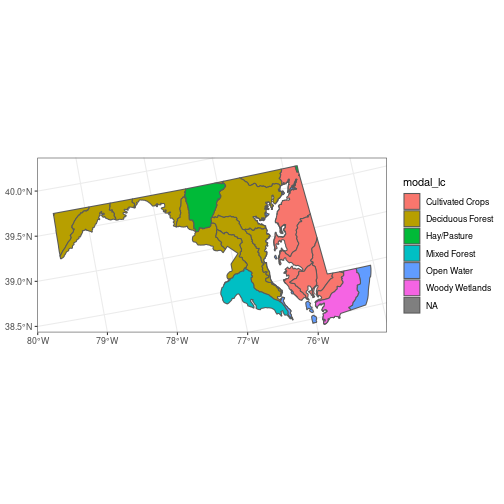Top of Section

## Interactive Maps

The mapview package is a great way to quickly view vector or raster data interactively, using a JavaScript library called leaflet. It produces interactive maps (with controls to zoom, pan and toggle layers) combining local data with base layers from web mapping services.

You can pass a vector or raster object to the mapview() function, which will create a map centered over your spatial object over a base tiled map.

By default, it imports tiles from Carto but you can switch to OpenStreetMap, ESRI World Imagery, or OpenTopoMap by clicking on the tile icon in the upper left.

library(mapview)

Warning: multiple methods tables found for 'crop'

Warning: multiple methods tables found for 'extend'

mapview(huc_md)


We are using all default arguments for mapview(), but there are many additional options that you can set. You can see a few of them in the next example. The map shows up in the “Viewer” tab in RStudio because, like everything with JavaScript, it relies on your browser to render the image.

Additional spatial datasets (points, lines, polygons, rasters) in your R environment can be added as map layers using +. Mapview will try to make the necessary transformation to display your data in EPSG:3857.

mapview(nlcd_agg, legend = FALSE, alpha = 0.5,
map.types = 'OpenStreetMap') +
mapview(huc_md, legend = FALSE, alpha.regions = 0.2)